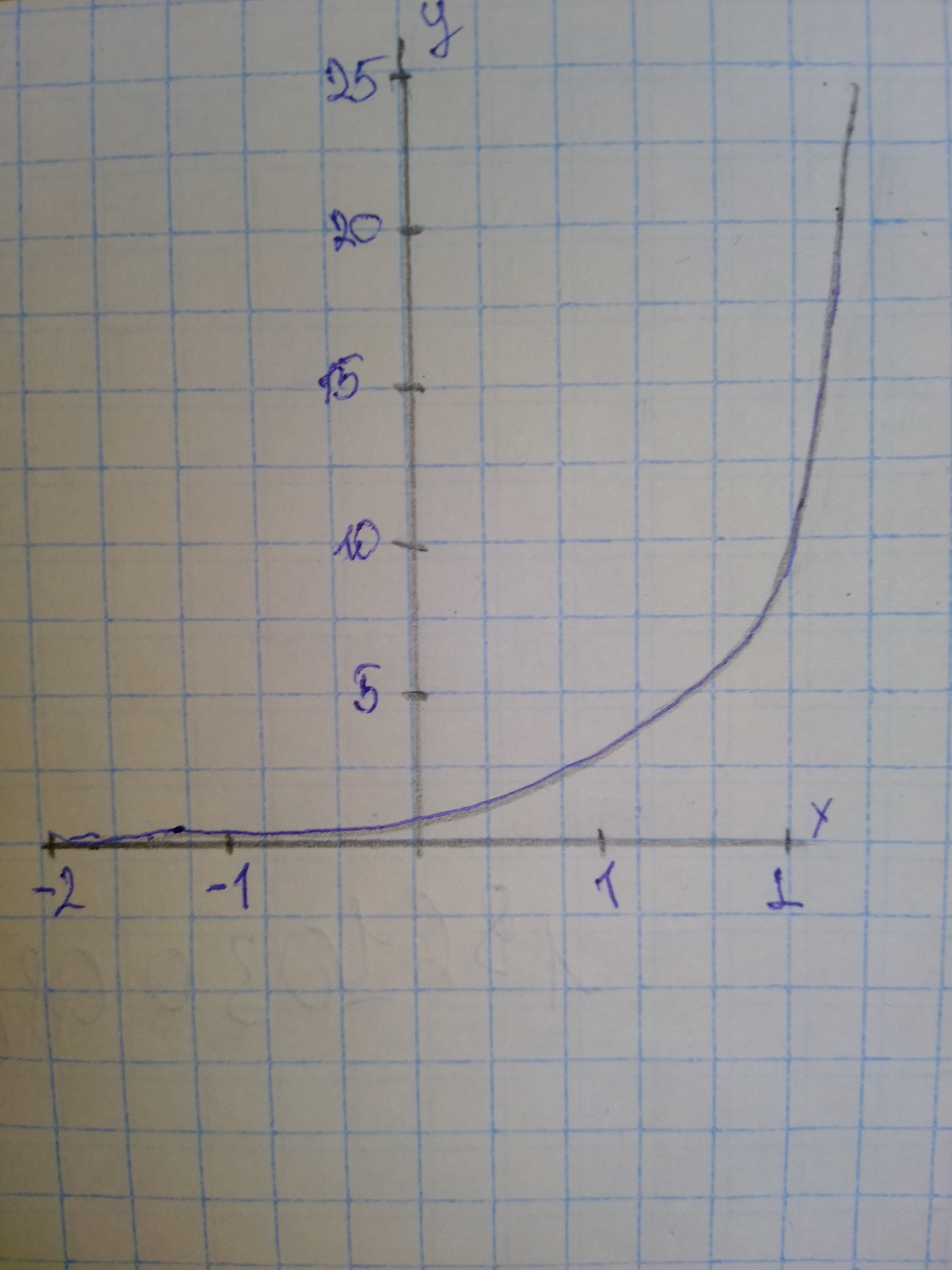# Tell whether the function represents exponential growth or exponential decay. Then graph the function. y=7^{x}Line 2020-12-28 Answered
Tell whether the function represents exponential growth or exponential decay. Then graph the function.
$y={7}^{x}$
You can still ask an expert for help

• Questions are typically answered in as fast as 30 minutes

Solve your problem for the price of one coffee

• Math expert for every subject
• Pay only if we can solve itLacey-May Snyder

Step 1
The base of the function , so the function represents exponential growth.
Step 2